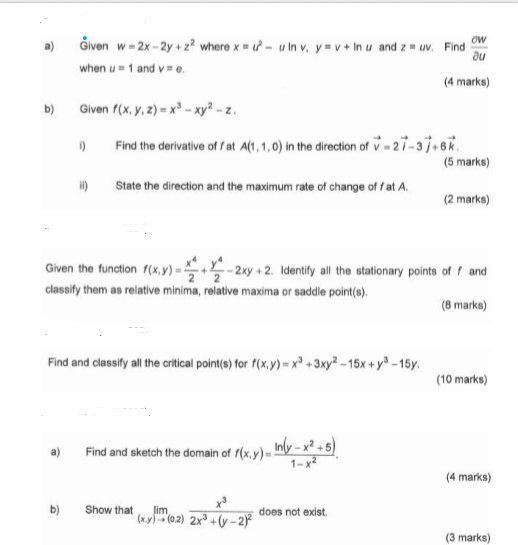### Create an Account

Already have account?

### Forgot Your Password ?

Home / Questions / ow au Given w- 2x - 2y + 2? where x1 - Inv, y v + In u and 2 - u. Find when u 1 and v. (4 ...

# ow au Given w- 2x - 2y + 2? where x1 - Inv, y v + In u and 2 - u. Find when u 1 and v. (4 marks) Given f(x, y, z) = x8 xy2 - . 1) Find the derivative of at A(1,1,0) in the direction of 27-37+67 (5 mar

ow au Given w- 2x - 2y + 2? where x1 - Inv, y v + In u and 2 - u. Find when u 1 and v. (4 marks) Given f(x, y, z) = x8 xy2 - . 1) Find the derivative of at A(1,1,0) in the direction of 27-37+67 (5 marks) 11) State the direction and the maximum rate of change of fat A. (2 marks) Given the function f(x,y) - -2xy + 2. Identify all the stationary points of and classify them as relative minima, relative maxima or saddle point(s) (8 marks) Find and classify all the critical point(s) for f(x,y) = xº + 3xy2 - 15x +y8 - 15 (10 marks) Find and sketch the domain of f(x,y) - Inby -x +5) (4 marks) Show that lim (xyl (0.2) 2x +(y-2) does not exist (3 marks)Apr 14 2021 View more View Less

#### Answer (Solved)Subscribe To Get Solution